Clutch Prep is now a part of Pearson
Ch. 1 - A Review of General ChemistryWorksheetSee all chapters

# Resonance Structures

See all sections
Sections
Intro to Organic Chemistry
Atomic Structure
Wave Function
Molecular Orbitals
Sigma and Pi Bonds
Octet Rule
Bonding Preferences
Formal Charges
Skeletal Structure
Lewis Structure
Condensed Structural Formula
Degrees of Unsaturation
Constitutional Isomers
Resonance Structures
Hybridization
Molecular Geometry
Electronegativity
Isomer
Polar Vs. Nonpolar
Covalent Bond
Polar Bond

Resonance is used to represent all the different ways that identical molecules can distribute electrons.

###### Rules for Drawing Contributing Structures
• Use curved arrows to represent electron movement
• Use double-sided arrows and brackets to link contributing structures to each other
• Arrows always travel from region of HIGH electron density to LOW electron density

The net charge of each structure must be equal

Concept #1: The rules you need for resonance:

Transcript

Resonance theory is used to represent the different ways that the same molecule can distribute its electrons. So what that means is that it turns out that even though the connectivity or how atoms are connected isn't going to change. The electrons between them can move sometimes and that's what resonance theory is all about.
So I need to show you some rules and you guys are going to get the hang of it as I go along. The first thing to know is that atoms will never, ever move. The reason is because, remember what I said, the connectivity of those atoms, how they're connected to each other, doesn't change. The only thing that changes is the kinds of electrons that are in between them that are keeping them linked together. The only thing that moves is the electrons.
When I talk about electrons, what I'm talking about is pi bonds, pi bonds move, and I'm also talking about lone pairs. What that means is that literally, I'm not moving any atoms, all I'm moving is double bonds around or triple bonds around and I'm also moving where lone pairs are at. That has to do with the electrons that are moving throughout the molecule.
Something about resonance structure is we're going to find out that there's something called contributing structures. Contributing structures are structures that both contribute to the actual representation of the molecule because they average together. And what we're going to find out is that none of these contributing structures are actually going to look like the actual molecules. What that means is the molecule is a blend of all the different possible resonance structures that a molecule can have.
Let's go ahead and learn some rules. First of all, we're going to use curved arrows to represent electron movement. Just so you know, these rules are going to apply to the rest of organic chem. We're going to keep using these rules any time that we're moving electrons which is pretty much all the time.
So what a curved arrow would look like is like this. Notice that I'm using a full arrow and I'm curving it around. What that means is that two electrons, that represents two electrons, are moving from one place to another.
What we're going to do is after we've built our resonance structures, we're going to use double-sided arrows and brackets to link related structures together. That means that once I figure out my resonance structures, I link them together using these double-sided arrows like I have here and then brackets like I have here.
Then finally, well not finally, but arrows are always going to travel from regions of high density, high electron density to low electron density. Like I said, this is a rule that applies for the rest of organic chem. Anytime we're moving electrons, we always start from the area of the highest density and move to the area of lowest density.
What that means is that, for example, a positive charge would be an area of low density because that means that you have electrons missing, right? So what that means is you would never start an arrow from a positive charge. In fact, you would always go towards the positive because that's the area of low density.
Then finally, the net charge of all the structures that we make must be the same. The reason for that is that remember that resonance structures are different ways to represent the same molecule and what that means is that all of them should have the same net charge because we're distributing the electrons different, but we're not adding any electrons or subtracting any electrons. What that means is they should really all have the same charge.

###### Common Types of Resonance

Concept #2: Common ways to move arrows in resonance.

Transcript

What I want to do now is I want to talk about common forms of resonance. These are patterns that I've basically just discovered while teaching organic chemistry and I want to share these with you guys. Let's talk about basically three right now. We're going to have cations, anions and then neutral heteroatoms.
Let's talk about cations first. Cations, the way that this works is if you have a cation next to a double, so let's go ahead and put that next to a double bond. What that gives us the ability to do is now to switch the place of those electrons because what I have is an area of high density on one side, which is a double bond. Remember that a double bond not only has a sigma bond, but also has a pi bond. Remember that pi bonds are extra electrons that are shared between two atoms so that's something that I can actually move. Then I have an area of low density which is my positive charge.
If I want to move this around, what do I do? Well, it turns out that this is kind of, this is one of the easier examples. If you have a positive charge and a double bond next to each other, you can actually kind of swing them open like a door hinge using one arrow, so what that means is I would start from the high density, my double bond, and I would move towards the positive charge. But I wouldn't make it just towards the positive, I would make it towards that bond.
So what I'm going to get now is that now I get a double bond in the place where the positive used to be and now my positive moves over here. What that means is that now by positive is actually distributed from that red, from the left side, over here on the red. Then over on the blue side, it's going to the right side as well.
So what I'm trying to say, is that anytime you have a positive charge next to a double bond, it can be represented by both of these drawings. It's not just going to stay in one place. Automatically, just by laws of chemistry, it's going to wind up switching places at some point.
The real molecule is going to look like an average of both of these or a combination of both of these. That's why I talked about the fact that none of them is a true representation. The best representation is by hybridizing both of these. I'm going to talk about what that is in a little bit.
Now let's look at anions. What if I had a negative charge next to that double bond. Now I have to ask you guys, what do you think is going to be the region of the highest electron density? It turns out that the double bond has a lot, but now that we have a full negative charge that's going to have even more electron density because the full negative charge means it just has a lone pair, just hanging out.
If we have a full negative charge, we're actually going to use two arrows. What I'm going to do is I'm going to take these electrons and push them into this bond making a double bond. But now we have an issue. If I make a double bond there, then we're going – let's look at this carbon right here. How many bonds did it already have? Well, it already had a double bond, that's two. It already had a bond to hydrogen, that's one. And then it already had a bond to carbon. So we had four bonds already.
If I make another bond with that negative charge, how many bonds is that carbon going to have? It's going to have five. That would be really, really bad. That would be basically impossible. You can't have a carbon with five bonds. That would be super terrible.
So this is a situation where we're going to use a rule that's called make a bond, break a bond. If I make a bond on this side, in order to preserve the octet of the middle carbon, I must break a bond because if I don't then I'm going to give this carbon, that I'm shading in green, I'm going to give it five bonds and that just sucks. If I make this bond, I have to break this bond. When I break that bond, what winds up happening is that now I get a negative charge over here.
As you can see with a positive charge, I didn't have to actually break any bonds because I was never violating any octets. But in the movements of anions or negative charges, I do have to break a bond because I am going to violate an octet. So what's important to note here is that cations move with one arrow and then anions move with two arrows. Not so bad, right?
Just remember that positive charges, they can swing like a door hinge, whereas with a negative charge, I'm going to use make a bond break a bond because of the fact that I have to preserve that octet of the middle atom.
Then let's look at neutral heteroatoms. This is the last situation, what this would be is that, remember that I said we can move double bonds and we can move lone pairs. So imagine that I have a lone pair here. Let's say imagine that I have two lone pairs there for that oxygen because remember that oxygen has a bonding preference of two bonds and two lone pairs. Cool.
Then imagine that the nitrogen has one lone pair because remember that the nitrogen has a bonding preference of three bonds and one lone pair. Remember this? This is how it's going to satisfy its octet and how it's also going to satisfy its valence.
Now let me ask you guys a question. Is there any way that I can turn these lone pairs, one of these lone pairs into a double bond and not break an octet? It turns out, let's look at our options. Basically, the two options are this. Either I could move one of these green lone pairs down here and make a triple bond or what I could do is I could move one of these red lone pairs here and make a double bond.
It turns out, you guys might be thinking, “Well, Johnny, why would I only move in that direction? Why couldn't I move like this? Why wouldn't I move the electrons down and make a double bond there?” Well, first of all, the reason is because double bonds and electrons are the things that usually switch places, so I would want to go in the direction that's going to go towards the double bond. I wouldn't want to go away from it. I would not go in this direction because that's away from my double bond.
But on top of that, check this out. I have a hydrogen here, right? If I move these electrons down into this area, I would make a double bond here. I'm sorry, I actually had more than one hydrogen. I had two. There's two hydrogens there because that's a CH2.
If I move these electrons in here and make a double bond, I'm going to break the octet down here and there's going to be no fixing that. There's actually no bond that I can break because these are all single bonds and you can't break single bonds in resonance theory. What that means is that I would wind up getting a double bond down here that would violate this octet and it would suck. That would not be a good resonance structure.
Instead, I'm going to deal with the other two situations that I was talking about, which is that either the O just moves down and makes a triple bond or the N lone pair jumps up and makes a double bond.
Let's look at the O making a triple bond. If the O made a triple bond like this. Let's look at this for a second. Don't draw it, just look at it. How many bonds would this carbon have? It would also have five, so we would break another octet by doing that. Is there any way that we can break a bond to make that carbon feel better? No, because it turns out that there's just single bonds on both sides, so there's nothing we could do. We would be stuck. We're definitely not going to move this lone pair either.
Now I have one last choice. The last choice is that I would move these electrons from the N up and make a double bond. If I make a double bond here, how many bonds will that center carbon have? Still five, so it looks like I'm screwed. Either way, I'm always making five bonds.
But there's one difference with this one. If I go ahead and go up and make the double bond up towards that carbon, guess what I can do? I can break a bond, so this is a situation where I'm making a bond towards a double bond. The reason that a double bond is helpful is because double bonds I actually can break, whereas single bonds, you're not allowed to break.
But double bonds, notice that I have these electrons in this double bond that are free to move. So what I'm going to do is I'm going to make a bond. And then for the sake of preserving the octet of this carbon right here, I'm going to break a bond and that would be right here.
What I would do is I would basically turn two electrons from that bond into a lone pair on the oxygen and that's going to preserve the four bonds that I need for that carbon right there because I'm making one, but I'm also breaking one.
What that would look like after I draw the resonance structure is I would now have a double bond here. I would have no electrons in the end because I just used those electrons to make the double bond. And then instead of having two lone pairs, now I would have the two lone pairs from before, so let's go ahead and draw those, the green ones. But now I'm going to have one more lone pair. The last lone pair comes from the bond that I broke because basically what I did is I took two electrons from that double bond and I made them into a lone pair.
Now all I have to do is count formal charges and we're done. This oxygen, it wants to have six electrons, but it turns out that it has seven. Because remember we're counting sticks and dots. So this would have a negative charge. The N wants to have five electrons total, but right now it just has four bonds. It has the double bond. It has the single bond there and then it has the hydrogen. That means that the nitrogen wants five, but it only has four, so it's going to have a positive charge. And that is our resonance structure.
So what we have effectively done is we've taken those lone pairs and we've redistributed them around and now we're showing another way that these electrons can exist in this molecule. But notice, that we're never moving single bonds. Single bonds are a big no-no. Don't break those. And also we're not rearranging the way that atoms are connected.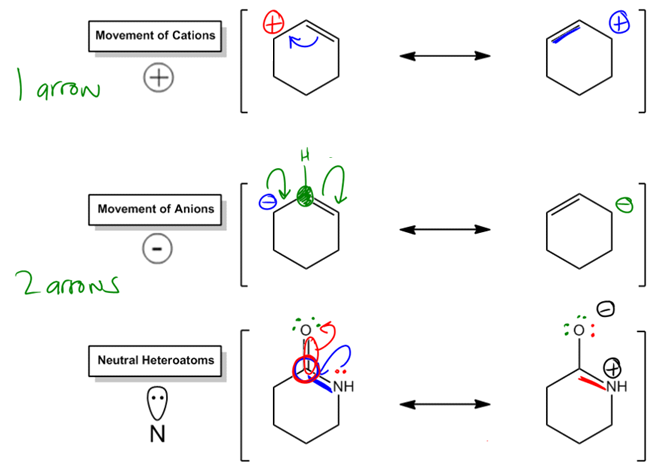Draw all of the contributing structures for the following molecules:Example #1: Draw all of the contributing structures for the following molecule.

Transcript

Alright, so there are a few things that you should remember that I told you guys were very important about resonance structures. First of all, remember that we use curved arrows. Easy. Remember that we use brackets with little double sided arrows to link structures. But also remember, that we always start from the area of highest electron density and work our way to the areas of less density.
In that case, that has to be the nitrogen because the nitrogen has a full negative charge on it. That means that is the most negative thing.
So what I want to do here is I want to try to move those electrons. The only way that I can move them is by becoming a double bond, or becoming a pi bond, I'm sorry.
So if these electrons move down here and became a pi bond, that would be great except I have a problem, if I did that then this carbon would have five electrons on it or five bonds. Five bonds is terrible. That would break the octet rule. It would be ten electrons, by the way. I'm sorry. Not five. Ten electrons. It would break the octet rule.
If I make that bond, what do I have to do? Do you guys remember? I have to break a bond. Why did I draw it at the bottom? You could have drawn it at the top too. But if you make a bond, you have to break a bond and this is that pattern that I told you guys that – oops that was weird – that anions come with two arrows. The reason is because anytime you're making that new double bond, you're going to have to break a bond as well or new pi bond.
What that's going to do is it's going to give me a structure that looks like this. We know I have N with a triple bond, carbon and then an oxygen. Now nitrogen already gave up one of its lone pairs to become a triple bond so that means it only has one lone pair left. Carbon has the same amount of electrons as before, it's just they're arranged a little differently. And then oxygen has one additional lone pair because the electrons from that double bond became a lone pair.
So basically the additional lone pair is this red one. And that red one came from this bond over here breaking. Does that make sense? Cool.
So now we have to do formal charges. I would have – it's funny that I put my negative there. I actually would have a negative right here on the O. And then would I have any other charges that I have to worry about? No. The carbon is fine and the N is fine.
Really, that's it. I just got my resonance structure. Are there any other things that we could do? Not really because if I make this negative. Let's say that I go back. I put this negative back here, once again I'm going to have to break a bond. What that means is that these two resonance structures are going to be basically two different versions of the way this molecule could look, but that's it. That's the only thing that it could do.
So I'm going to put brackets around this and that's going to be a wrap. That's going to be the end of that problem.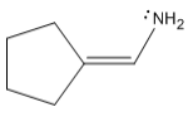Example #2: Draw all of the contributing structures for the following molecule.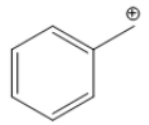Example #3: Draw all of the contributing structures for the following molecule.

###### Resonance Hybrids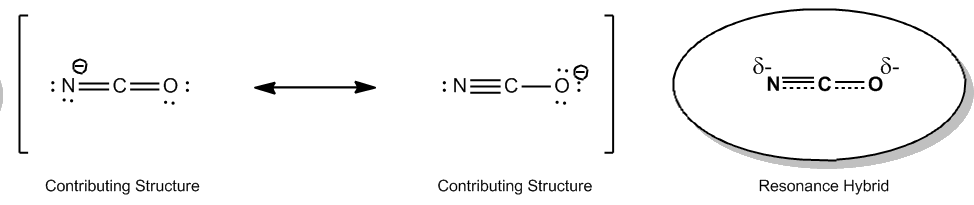Concept #3: How to draw a resonance hybrid.

Transcript

Alright guys so we just talked about resonance structures and how one single molecule could have several different contributing structures, that's what we call each structure that has a slightly different distribution of electrons we call that a contributing structure, well it turns out now what we want to talk what is hybrids how they blend together and also which will be the major structure in terms of which will represent the way that the molecule looks the most so let's go ahead and begin, so basically the resonance hybrid is going to be a mathematical combination of the contributing structures, OK? And what it does is it indicates where the resonating electrons within a molecule are most likely to reside so what we do for this is we literally combine the two different resonance structures into one drawing or two, three, four etc. and we combine them all into one drawing and then we try to analyze which will be the resonant structure that would contribute the most of that hybrid, so here is a more sure that we're going to deal with a lot in *Orgo 2* it's called Isocyanate and I don't really care that you guys don't know that much about it but what's interesting is when we take a look at the contributing structures here so for one of these I have two double bonds and what I've done here is I've done I've used the negative charge rule to make a bond break a bond so what I'm doing here is I'm taking these electrons here, making a triple bond but then if I made that triple bond that carbon would violate its octet and it would have five bonds so that it's going to break this bond and make a negative charge over there so at the end what I'm going to get is two different structures, one that has a negative charge on the N, one that has a negative charge on the O, OK? what the resonance hybrid is it's a blend of both of these so what I would do is I would just draw the parts of the bond that are not changing so for example notice that here I always have at least two bonds between the carbon and the nitrogen, in this structure I actually have three bonds but in this one I have two so I would draw those two, OK? On the Oxygen side I always have at least one bond between the carbon and the oxygen but in this case, I have 2, OK? Then what I would do is I would draw partial bond from the nitrogen to the carbon and from the carbon to the oxygen what that indicates is that this bond is being created and destroyed at the same time, OK? what it also indicates is that basically I'm in between both, OK? And then finally I put partial charges in all the places that have a negative charge, Why? because the hybrid like I said it's not an equilibrium this is not like, OK this is not like what we talked about in Chem 2 where the reaction that favors the right or favors the left and it goes back and forth, No...What this is it's a mathematical concept where I say, OK this gets let's say 40 percent of the molecule this is 60 percent and the actually molecule looks like a blend of both of them does that kind of make sense? So, in this case I've drawn my hybrid, notice that basically everything that's changing is showing on this hybrid I'm showing that the bonds are being broken and destroyed broken or broken and created at the same time and I'm also showing that the negative charges moving from one place to another, OK?

The hybrid is the drawing of the mathematical combination of all contributing structures. Remember, you can never break single bonds!

Which of these structures looks the most like the hybrid?

###### Major Contributors

Concept #4: How to determine which structure is most stable.

Transcript

Now in terms of major contributors, that's when we determine, okay, which of these is the one that looks the most like the hybrid? Is it number one or is it number two? To figure that part out, we have to use just a few rules.
Often, it turns out that one of the resonance structures will be more stable. So it turns out, let's say you have more than one resonance structure, one of them is the most stable. So that's going to be the one that we use. That means that it's going to contribute to the hybrid more than the others will.
And major contributors will often have the following characteristics. The first thing is that neutral structures are almost always going to be more stable than charged ones. If I have a choice between, let's say I have a resonance structure that's neutral and a resonance structure that has charges on it, I'm going to pick the neutral one to be my major contributor and to be the one that looks most like the resonance hybrid. Why? Because that's the one that's overall most stable.
Even if the other one is possible, it may exist to some extent, but the one that's really going to exist in excess, or not exist, but the one that's going to contribute in excess is going to be the neutral one. Does that make sense? Cool.
So a good example for that would be where I showed you guys the neutral heteroatom example on the other page, where there was one that had basically a neutral structure and then one that had a positive and a negative. The major contributor would be the one that was just fully neutral. The one that had a positive and a negative would be a minor contributor because that one already has charges. It's not as stable.
Let's keep looking at this. Another rule is that, if possible, every atom should fill its octet. What that means is that we're going to look towards resonance structures that are not satisfying the octet. Let's say ones that have too few electrons. Those are usually going to be minor contributors. If I have a choice between a resonance structure that fulfills all of its octets and one that doesn't, I'm always going to pick the one that does fulfill its octets.
And then finally, the electronegativity trends are going to determine the best placement of charges. Now I know it's been a really long time since you talked about electronegativity. In fact, for a lot of you guys, you haven't heard about it since gen chem.
I just want to remind you guys that this is the electronegativity scale. What it basically says is that as you go the right and as you go up, your electronegativity gets higher. What that means is that fluorine is the atom that is most comfortable having a negative charge or having electrons on it.
If I were to pick that the negative charge is on a fluorine or the negative charge is on a carbon, which one is going to be more stable? What do you guys think? The fluorine, right because that's electronegative. That means that it likes to have electrons or negative charges on it, whereas carbon is not as to the right as fluorine, so carbon is going to be a lot less comfortable having that negative charge. Does that make sense?

Often one of the resonance structures will be more stable, so it will contribute to the hybrid more than the others.

Use the octet rule and electronegativity trends to determine the best placement of charges. (i.e. Fluorine is more stable with a negative charge than oxygen).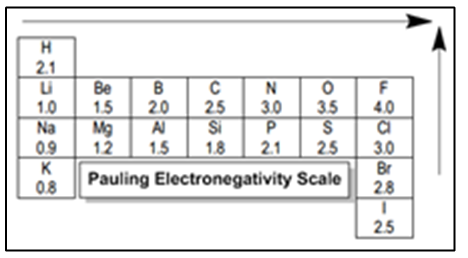Example #4: How to determine which structure is most stable.

Transcript

Alright guys, so it turns out that there were no neutral structures, so I couldn't use the neutral rule. All of these molecules fulfilled their octets, so I couldn't use the octet rule. But, we had differences in electronegativity.
It turns out that the O, being with a negative charge, is going to be more stable. That would be my major contributor. Why? Because it turns out that O is more electronegative, I'm just going to use EN for electronegative, than nitrogen. What that means is oxygen is more comfortable having that lone pair on it than nitrogen is. So this would be less electronegative. Because of that, this is going to be the minor contributor.
When I go ahead and draw my resonance hybrid, we can draw it the same exact way, but we have to acknowledge, let's say I'm drawing it like this N, C, O, partial bond, partial bond. One of the ways that we could draw this is we could draw the partial negative on the O bigger. So we'll draw bigger partial negative on the O and a smaller partial negative on the N.
Why is that? Because remember, we just said that even though both of these coexist, the negative on the O is going to be the most stable. That means that most of the time it's going to look more like this one. Sorry, that kind of got blurry. More like this one and less like the other one. This means that my hybrid would be a bigger share of the major contributor. Does that kind of make sense?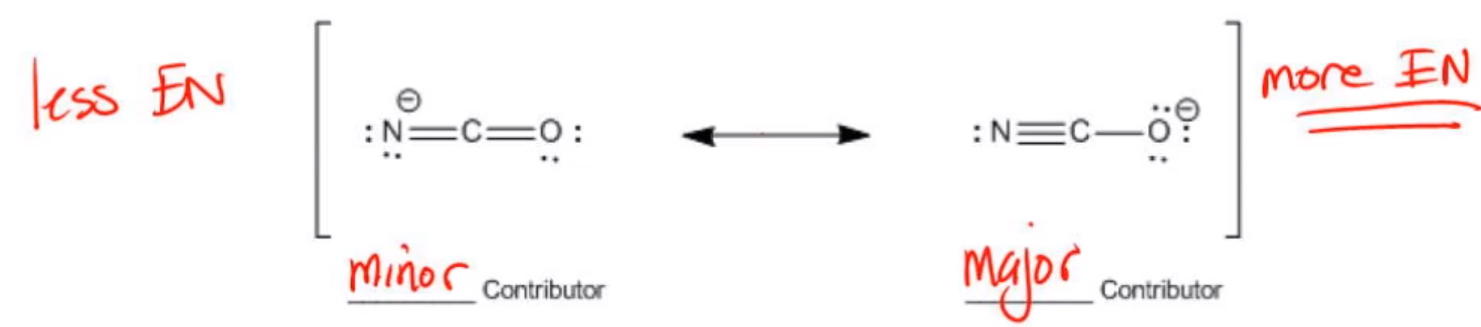By applying the rules we learned to the above example, we saw that the negative charge could either rest on the nitrogen or on the oxygen. Since oxygen is more electronegative, that structure is the major contributor.

Let's practice by drawing all of the contributing structures for the following molecules. Label the major contributor if applicable and draw the resonance hybrid.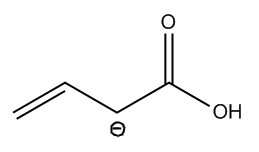Example #5: Draw all of the contributing structures for the following molecule. Label the major contributor if applicable and draw the resonance hybrid.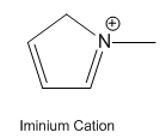Example #6: Draw all of the contributing structures for the following molecule. Label the major contributor if applicable and draw the resonance hybrid.

Great job! Not the easiest of topics but we got through it!